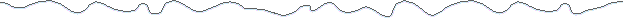Off-Axis Field Due to a Magnetic Dipole MomentAt points far from a magnet, solenoid, current loop or Helmholtz coil, you can approximate the field by modeling the coil (or magnet) as a magnetic dipole moment. The error of this technique is less than roughly 2% at distances greater than 5 times the largest dimension of the coil (or magnet), and it approaches 0% as the distance approaches infinity.B is the magnetic field, in teslas, at any point in space that isn't at the origin. It is equal to the sum of two field components,

BX the magnetic field component which is aligned with the x axis and

Br the magnetic field component which is in a radial direction.

x is the distance, on axis, from the dipole to the field measurement point, in meters.

l is the distance from the dipole to the field measurement point, in meters.

q is angle between the dipole axis, and the position vector of the field measurement point.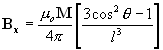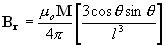where,

M is the strength of the magnetic dipole moment, in ampere meters squared (Am2).

m o is the permeability constant (1.26x10-6 H/m or 4p x10-7)

As always, units of linear dimension (l) and magnetic field (B) are adjustable by using an appropriate value for the permeability constant.Magnetic Dipole Moment

A magnetic dipole moment is a simple mathematical representation of a coil, electromagnet or permanent magnet ("refrigerator magnet") that can be used to predict the magnetic field at a distance from its source. The prediction is generally accurate only if the distance to the measurement point is much greater than the largest dimension of the magnet.

For example, the error is roughly less than 2% for distances greater than 5 times the largest magnet dimension.

For air core electromagnets,where,

N is the number of turns of wire in the magnet,

i is the current in the coil, in amperes, and

A is the mean area of the coil, in meters squared (m2).

For permanent magnets, magnetized in a single direction,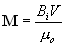where,

Bi is the "intrinsic induction" of the magnet, in teslas,

V is the volume of the magnet, in meters cubed (m3).

How do I figure out what Bi is?

For many fully magnetized rare earth (Neodymium Iron Boron or Samarium Cobalt) ceramic or ferrite magnets, Bi is approximately equal to Br, which is available from the magnet manufacturer. For Alnico magnets, Bi is approximately equal to Br only if the magnet's length to width ratio (magnetized lengthwise) is much greater than 1.

Typical values for Br are:

0.4 teslas for ceramic or ferrite magnets,

1.0-1.5 teslas for Alnico and rare earth magnets.Predicting the field due to a dipole moment based on a single field measurement on axis.

If you can make a single, on axis, field measurement at some distance (greater than 5X magnet size) from a magnet, then you can predict the field at any point in space surrounding the magnet.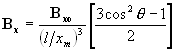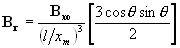where,

Bxo is the measured field strength at some point,

xm meters from the center of the magnet and on its axis.

 Magnet Formulas web site, © 2005 by Eric Dennison. I am developing an updated and expanded version of the magnet web site and encourage you to visit this work in progress and comment on what you see. As a self-employed software engineer trying to balance time between work and magnets, your encouragement in building this site is always welcome! Please consider clicking the PayPal link and making a contribution to support the maintenance and development of this site. Thank you for visiting!